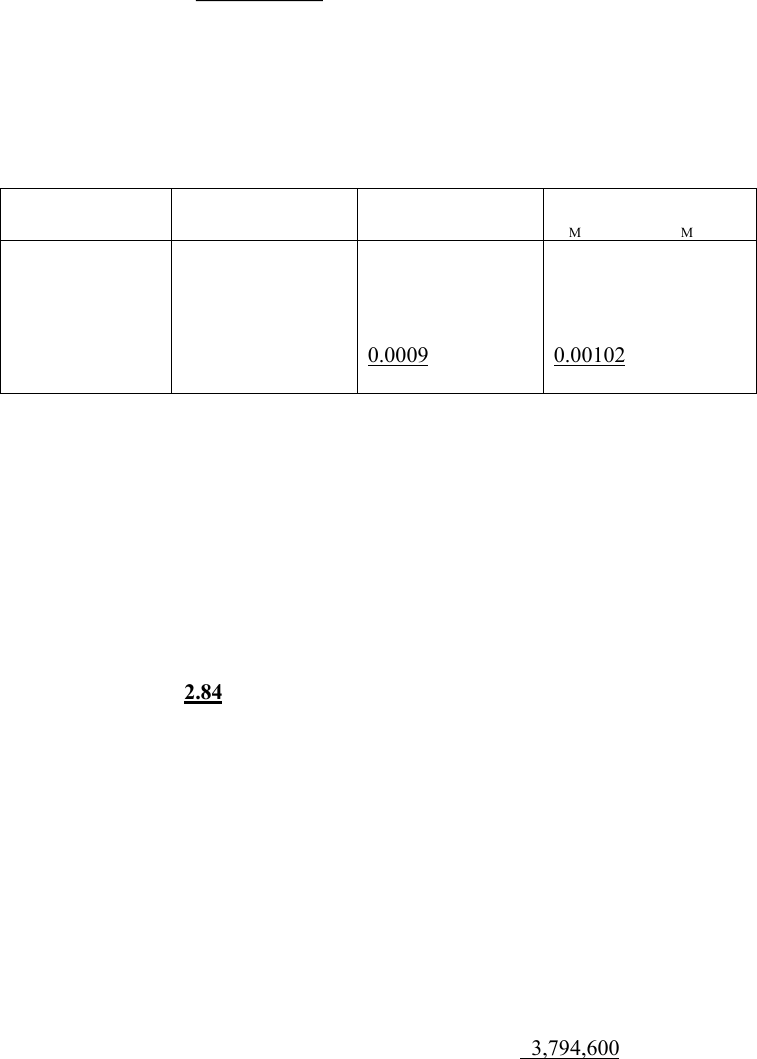# 33:390:400 Lecture Notes - Lecture 5: Capital Surplus, Systematic Risk, Net Present Value

29 views4 pages
School
Department
Professor1
Corporate Finance
Problem Set #1-1 Solutions
#1. D/E=0.25 D/(D+E) = 0.2 E/(D+E) = 0.8
WACC = [.8 x .12] + [.2 x .07 x (1-0.4)] = .1044 or 10.44%
=+
+=
5
1t )1044.01(
million \$8
million \$30 - NPV t
= - \$11,535 Reject the project
#2.
(a) average rD = (-0.05 + 0.05 + 0.08 + 0.15 + 0.10)/5 = 0.066
average rM= (-0.12 + 0.01 + 0.06 + 0.10 + 0.05)/5 = 0.02
(b)
rD - average rD
rM - average rM
(rM - average rM)2
(rD - average rD) x
(rM - average rM)
-0.116
-0.016
0.014
0.084
0.034
-0.14
-0.01
0.04
0.08
0.03
0.0196
0.0001
0.0016
0.0064
0.0009
0.0286
0.01624
0.00016
0.00056
0.00672
0.00102
0.02470
Estimated beta for Douglas= 0.02470/0.0286 = 0.864
#3.
(a) We first unlever the equity beta of the comparable firm using the formula,
E = (1+(D/E)(1-Tc)) x unlevered
1.80 = (1+200/400 x .80) x unlevered
unlevered = 1.29
Since two firms have the same level of business risk, the asset beta for Firm A would be 1.29 as well.
Now we use the same formula again to estimate equity beta of Firm A.
E = (1+1.50 x .80) x 1.29 = 2.84
(b) Equity beta is higher for Firm A than for the comparable firm. The difference is because Firm A has a
higher debt ratio and therefore has higher financial risk than its comparable firm. If a firm has larger
amount of debt, it has to make larger debt payments. Since these debt payments are fixed costs, cash
flows to shareholders (or stock returns) of firms with larger debt payments would be more sensitive to
the market returns. Thus the systematic risk of these firms would be higher and their equity beta
should also be higher reflecting the higher systematic risk.
#4.
(a) Shareholders’ equity
Common stock (\$5 par value; authorized 500,000
shares; issued and outstanding 325,000 shares)
\$1,625,000
Capital in excess of par*
195,000
Retained earnings**
3,794,600
Unlock document

This preview shows page 1 of the document.
Unlock all 4 pages and 3 million more documents.

## Document Summary

Wacc = [. 8 x . 12] + [. 2 x . 07 x (1-0. 4)] = . 1044 or 10. 44% 1044 t = - ,535 reject the project. 0. 0286 (rd - average rd) x (rm - average rm) #3. (a) we first unlever the equity beta of the comparable firm using the formula, Since two firms have the same level of business risk, the asset beta for firm a would be 1. 29 as well. Now we use the same formula again to estimate equity beta of firm a. E = (1+1. 50 x . 80) x 1. 29 = 2. 84 (b) equity beta is higher for firm a than for the comparable firm. The difference is because firm a has a higher debt ratio and therefore has higher financial risk than its comparable firm. If a firm has larger amount of debt, it has to make larger debt payments.

## Get access